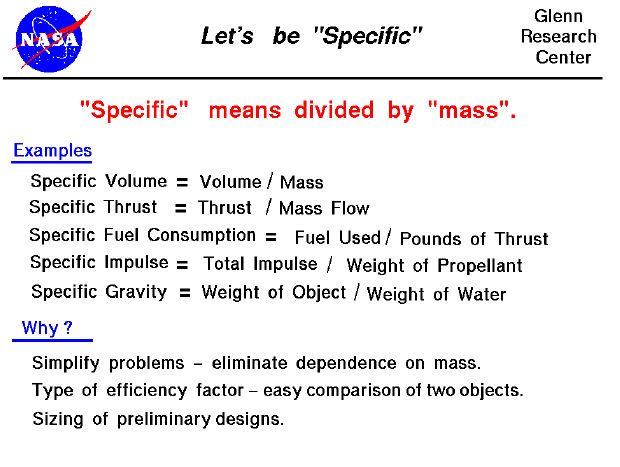In the study of propulsion systems, we often encounter the term "specific", as in specific fuel consumption, specific thrust, or specific impulse. Specific is a scientific term meaning "divided by weight or mass", You have probably encountered other "specific" terms in physics and chemistry. The specific volume of a gas is the volume of the gas divided by the mass of the gas contained in the volume. Specific gravity is the weight of an object divided by the weight of the same volume of water. Specific heat is the calories per weight of substance.

Why are we interested in "specific" properties? First, it simplifies our mathematical analysis of some problems by removing the dependence on mass. For example, the equations for the thermodynamic work of a gas contains the mass flow rate as a multiplier. We could carry it along throughout the analysis, but since the mass flow rate is a constant, the math is easier if we just divide it out at the beginning and put it back in at the end. Second, specific properties are an indication of efficiency. For example, two different engines will have different values of specific thrust. The engine with the higher value of specific thrust is more efficient because it produces more thrust for the same amount of airflow. Third, specific properties give us an easy way to "size" a preliminary design. For example, the result of a thermodynamic analysis is a certain value of specific thrust. The aircraft drag will define the required value of thrust. Dividing the thrust required by the specific thrust will tell us how much airflow our engine must produce and this determines the physical size of the engine.